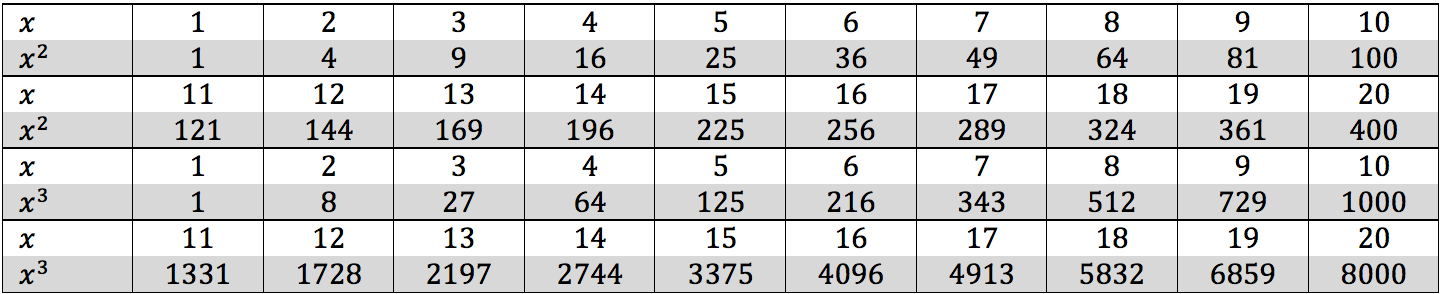Powers or exponents refer to multiplying the same number to itself a certain number of times, and the same is true for variables and algebraic expressions. The exponent will be located in the upper right hand corner next to the number and will be much smaller than the number (called superscript).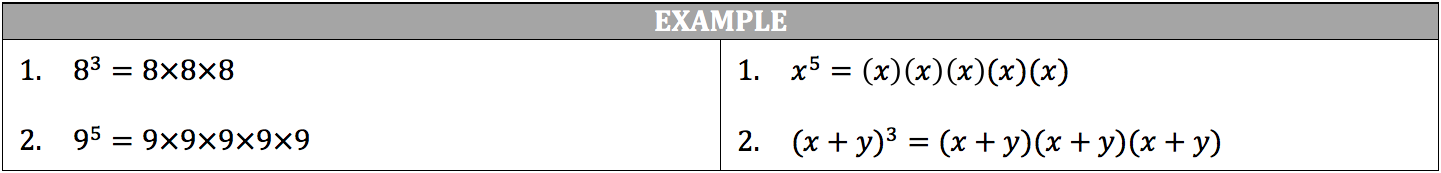When the power is represented as a positive integer, you simply multiply the number times itself the number of times designated by the power. When the power is represented as a negative integer, you take the reciprocal of the number and multiply the reciprocal times itself the number of times designated by the power. The negative in powers does not mean that the product will become negative; the negative only means you take the reciprocal of the integer. You may also take the number to its power first and then find the reciprocal of that result.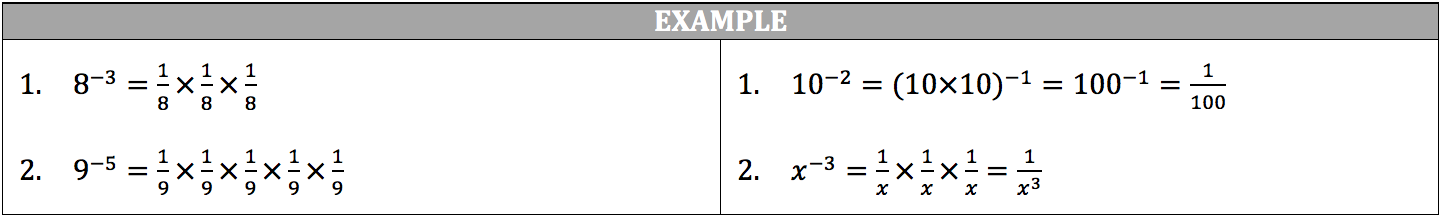Any fraction or decimal taken to a power that is a positive integer will always equal a smaller number. Any fraction or decimal taken to a power that is a negative integer will always equal a larger number. Any number taken to the power of one will equal the original number. Any number taken to the power of zero will equal 1.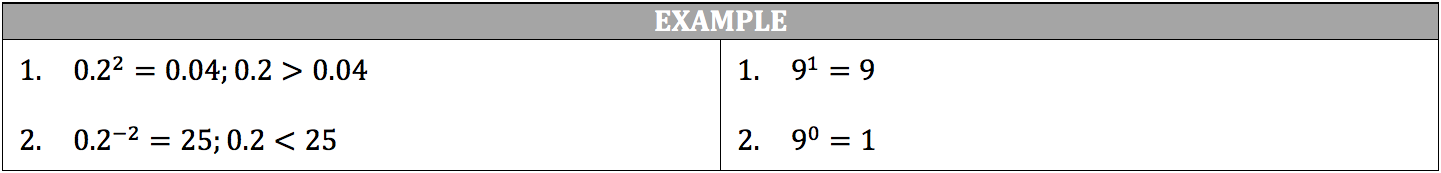When multiplying similar numbers with powers (negative or positive), such as 92 with 93, you add the powers together. When dividing similar numbers with powers (negative or positive), you subtract the powers. Remember, when you divide another number by a fraction, you may multiply the number by the reciprocal of the fraction to achieve the correct answer. When you take a number with a power to another power (negative or positive), you multiply the two powers together.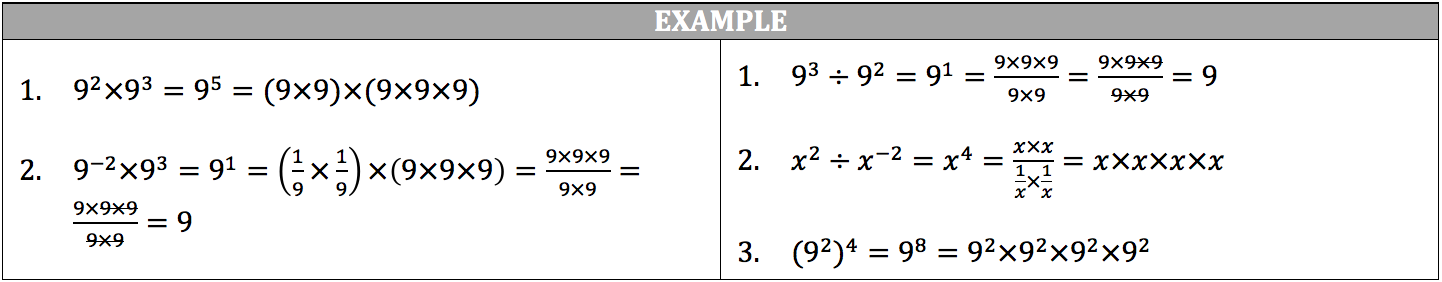Roots take the opposite action of powers, in that the root of a number is another number multiplied by itself a certain number of times to make the original number, such as 8 is the square root of 64 and 4 is the third root of 64.  The root can be written as the symbol √ (called a radical) and will encompass the original number. You can find the root of a number through factoring.  If you are looking for the square root of a number, you look for the same number multiplied to itself twice with no remaining numbers in the factor tree.  If you are looking for the third root of a number, you look for the same number multiplied to itself three times with no remaining numbers in the factor tree (and so on).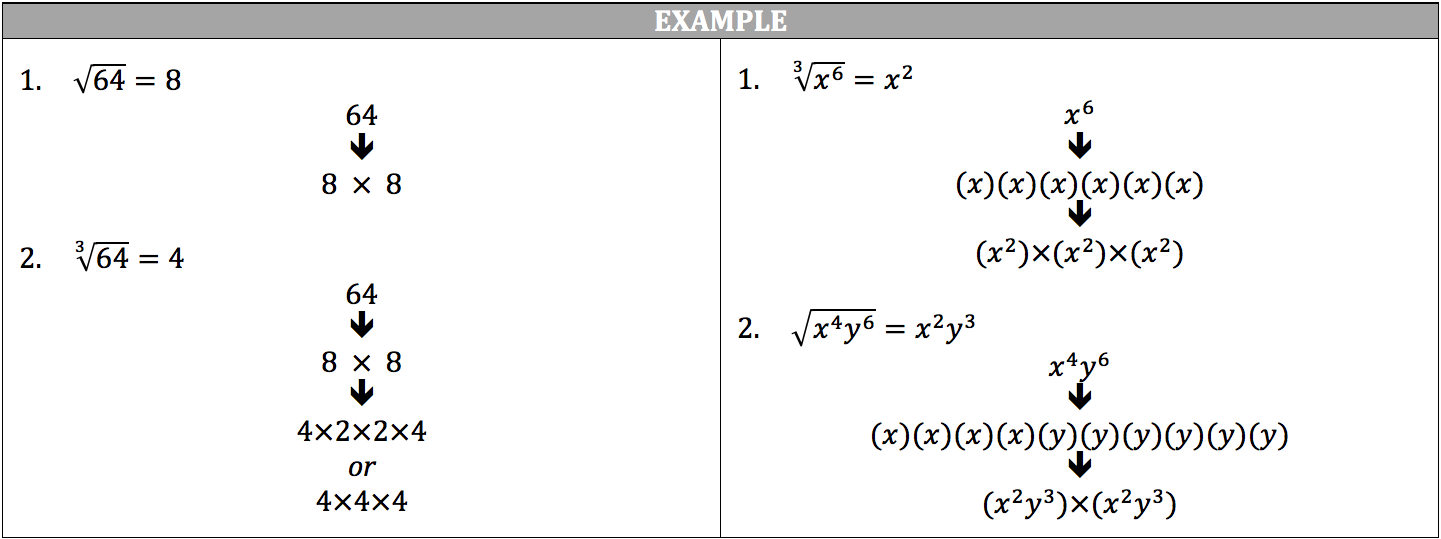Powers and roots may be represented together in a single fraction, where the numerator is the power and the denominator is the root: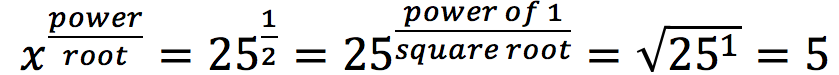When multiplying similar numbers with fraction exponents, you add the fraction exponents as you would normal fractions. When dividing similar numbers with fraction exponents, you subtract the fraction exponents as you would normal fractions.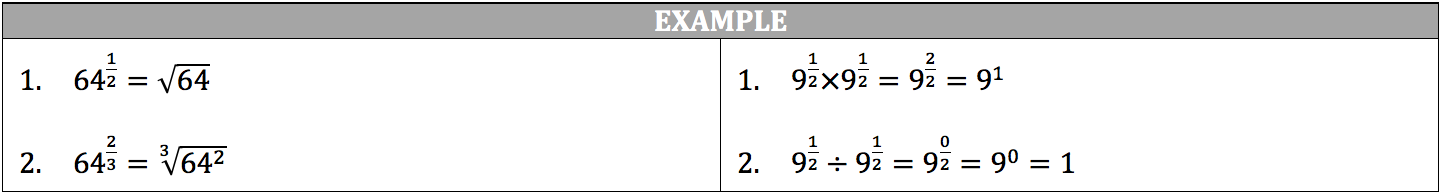Keep in Mind:

• A negative number taken to a power that is an even integer will result in a positive number; therefore, even roots will always have a positive and a negative solution. √81 = ±9; 9×9 = 81 and -9 × 9 = 81
• A negative number taken to a power that is an odd integer will result in a negative number.
• The even root of a negative number is an imaginary number.

Review this table of squares and cubes up to 20: